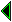## Criticism and reconstruction

The process of formalization must also incorporate a critical aspect when the need arises. Certain concepts which do not admit a satisfactory formalization may have to be jettisoned, modified, or relegated to the status of heuristic aids. It is hoped that this procedure leads only to superficial notational rigorization of mathematics, rather than the mutilation of important ideas and principles.

In a computer-based system especially, it's vital to avoid all notational ambiguities. Generally, the more flexible grammatical rules are, the more complicated they are too, and the more scope they leave for misunderstanding. A balance needs to be struck. Computer programming languages and formal specification notations have already blazed the trail, and we can look to them not only for warnings about the difficulties, but for solutions to them. For example, most people would agree that different precedences for binary operators are a good idea, but sometimes the precedences chosen can confound people's expectation. (In C the bitwise AND operator ' &' binds less tightly than the equality relation ' ==', a historical accident that must have caused innumerable programming errors.) A controversial question is the overloading of operators, e.g. the use of + for addition of both integers and floating point values. Possibly the theory of type classes [wadler-blott], intended to put overloading for programming languages on a firmer footing, contains lessons for formalized mathematics too.

A good example of abuse of notation in mathematics books is the use of f(x) to represent both application of a function f to an argument x, and the image under f of a subset, x, of f's domain. These ambiguities are often avoided by, for example, the use of upper case letters for sets and lower case for elements. But in pure set theory, there are often so many layers of sets that this sort of convention cannot be maintained; some writers, e.g. [kunen], carefully use f'x and f"x respectively. Similarly, f-1 is sometimes used to denote the inverse function, and at others merely reserved for the preimage under f, which may make sense even if there is no inverse function (left or right). Although when f is bijective these are equivalent, fairly sophisticated use of context is required to determine that.

Another notation that is usually clear intuitively but hard to describe formally is indexed set abstraction { f(x) | P[x] }, for example { (m,n) | m + n = p }. In general, it's not easy to determine whether variables are supposed to be bound by the construct or not (for example, the above example is probably intended to bind both m and n, but if m means something outside, that might no longer be so.) For this reason the Z specification language uses a more precise notation which separates the variables being bound and the condition which the tuples of variables need to satisfy. Mathematics contains other confusions between free and bound variables and between constants and variables. There is a popular disclaimer attached to the general quartic equation: 'a x4 + b x3 + c x2 + d x + e where e is not necessarily the base of natural logarithms'. And we have already discussed the problems of the Leibniz derivative notation. Another popular abuse of language is to employ X to refer to the poset (X,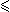), leaving the ordering implicit. [bourbaki-sets] has already shown a good way out here: we can take the ordering relation as primitive, replacing the reflexivity property in the usual definition of partial order by xy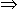xx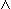yy, recovering X if desired as { x | xx }.27

It is quite possible that the critical faculties of the formalizer may go well beyond cleaning up sloppy notation. The formalizer may not merely want to reconstruct existing mathematics in a formal framework, but may prefer to develop a new (better?) mathematics. It is clear that Zermelo had the former aim in mind when he introduced his set theory,28 but not all logicians and philosophers of mathematics take such a respectful view of existing practice. In particular, among many mathematical logicians there is a strong tendency to be:

1. Constructivist --- although Brouwer had a visceral dislike of formal logic, constructivism is nowadays mainly the interest of logicians and computer scientists, not 'mainstream' mathematicians.29

2. Minimalist --- logicians are interested in discovering extremely simple systems in which a reasonable amount of mathematics can be done. For example, [takeuti-uses] has shown in detail how to reduce much of real and complex analysis and analytic number theory to a conservative extension of PA, and [fitch-book] has claimed that his provably consistent set theory is sufficient for most applications of mathematics in physics.

3. Sceptical --- perhaps because of the difficulty of finding consistency proofs, many logicians are apt to be wary of strong systems, even ZF set theory. Actually there are those who do not accept as obvious the consistency of Peano arithmetic, and some ultra-finitists who even find a belief in arbitrarily large numbers to be philosophically dubious.

Perhaps the formalization of mathematics provides an ideal opportunity to make a clean break, just as when one rewrites a program in a new language, it's a good opportunity to make some revisions. On the other hand, if we hope to interest many mathematicians, we need to accommodate existing mathematics. We are already trying to wreak one revolution by making the mathematicians formalize their work. Surely one revolution at a time is enough! And, if we take a crude measure of productivity, it seems much easier to develop classical mathematics than constructive mathematics. Formalization is not a mechanical and trivial process, but a significant research problem in itself. If we add to that the new problems which arise, say, when trying to constructivize mathematics, then we are probably going to find the process particularly difficult. Experience of Nuprl researchers indicates that formalization of constructive analysis and algebra is difficult, since the existing treatments leave a lot unsaid. Sometimes the textbook approaches do not scale up well in practice; for example, maintaining the standard Bishop 1/n bound on convergence of Cauchy sequences is very tedious when doing nontrivial things with constructive reals (like solving differential equations). But on the positive side, this can be seen as an interesting motivation for new research! For example, several practitioners of constructive mathematics consider G. Stolzenberg's approach to analysis much more suitable as the basis for computer formalization.30

Of course to some extent classical and constructive mathematics can coexist. The approach of [bishop-bridges] to constructive analysis has the merit of being compatible with classical analysis. But it is more complicated: one classical theorem often splits into several constructive versions (classically equivalent); the classicist may regard this as meaningless clutter. And certain constructive systems are actually in conflict with classical analysis and with each other --- [bridges-richmann] give an interesting comparative survey. So though it sounds very nice to say 'if a theorem can conveniently be proved constructively, then prove it constructively, and it can still be used classically', the real situation is more complicated. Those without constructive interests are unlikely to make the additional effort, anyway.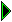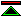John Harrison 96/8/13; HTML by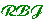96/8/16